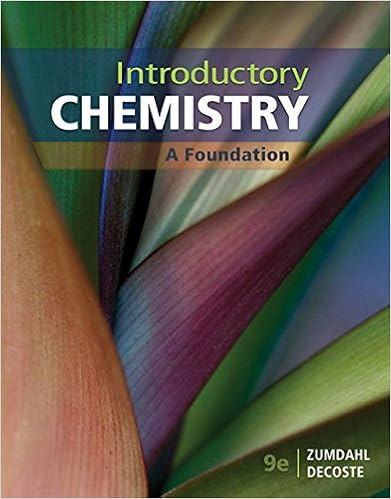# acid_base_practice_free_response_quiz (1).doc - Chapter 14...

• Test Prep
• 4

This preview shows page 1 - 4 out of 4 pages.

##### We have textbook solutions for you!
The document you are viewing contains questions related to this textbook.The document you are viewing contains questions related to this textbook.
Chapter 16 / Exercise 67
Introductory Chemistry: A Foundation
ZumdahlExpert Verified
Chapter 14 Practice Free Response I suggest you take this as a practice quiz! STUDY FIRST! Then try this! 1. Name/give formula for the following as acids or bases H 2 CO 3 ______________________________________ HClO _____________________________________ Hydroiodic acid _______________________________________ HNO 3 _________________________________________ HCN ___________________________________________ H 2 SO 3 ____________________________________ HClO 4 _______________________________________ Calcium hydroxide ________________________________________ H 2 S ___________________________________ 2. Put a * in front of the names/formulas of the strong acids/bases listed above. 3. Circle the stronger acid in each pair: a. HClO 2 or HClO 3 c. H 2 SeO 4 or H 2 TeO b. HI or HBr d. H 2 PO 4 - or HPO 4. a.) Name the acid: CH 3 COOH __________________________________ b.) Draw the structure of this acid. c.) Give the name of the functional group of this acid and circle the functional group in the structure you drew above. __________________________ d.) Write the balanced equation for the reaction of this acid with water. Label all species as Bronsted-Lowry acids and bases. : 4 4 -2
##### We have textbook solutions for you!
The document you are viewing contains questions related to this textbook.The document you are viewing contains questions related to this textbook.
Chapter 16 / Exercise 67
Introductory Chemistry: A Foundation
ZumdahlExpert Verified
5. Identify the Bronsted-Lowry acids and bases in the equations below. Place an A under the acids and a B under the bases. Draw a line connecting the conjugate pairs. C 6 H 5 NH 3 + + OCl - C 6 H 5 NH 2 + HOCl
•••